# Light rays bouncing in twisted tubes

Imagine a smooth curve $c$ sweeping out a unit-radius disk that is orthogonal to the curve at every point. Call the result a tube. I want to restrict the radius of curvature of $c$ to be at most 1. I am interested in the behavior of a light ray aimed directly down the central axis of one end of the tube, as it bounces with perfect reflection from the interior wall of the tube. This could be viewed as a model of an optic fiber, although I want to treat the light ray as a billiard ball and not a dispersing wave.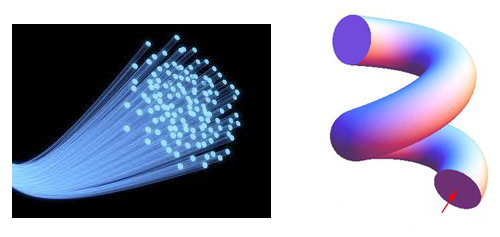Q1. Does the light ray always emerge from the other end?

I believe the answer is Yes, although there can be close calls:I would be interested in a succinct, convincing proof (or a counterexample!). Perhaps I should stipulate that if the ray hits a boundary singularity (as it nearly does above), it dies; otherwise it could pass through the center of curvature and reflect to its own reversal.

Q1 Answer: Yes if $c$ is $C^\infty$ (Dimitri Panov), Not necessarily if $c$ is $C^2$ (Anton Petrunin).

I explored one particular, maximally convoluted, snake-like tube, composed of alternating semicircles of radius 2 (so the central curve $c$ has curvature 1):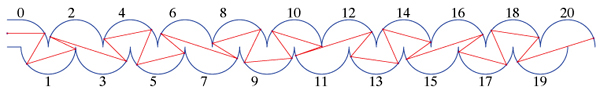The lightray behaves seemingly chaotically, although when I look at the angles the rays make with the $(+x)$-axis, there is a striking distribution. Here is a histogram for a tube composed of 1000 semicircles:Q2. Can you offer an explanation for the observed distribution of ray orientations? Why is the angle $\pm 17^\circ$ so prominent, and there are no ray angles whose absolute value lies within $[19^\circ,111^\circ]$? There are approximately 1.55 ray bounces per semicircle: Why? Is this approaching $\frac{3}{2}$? Or $\frac{\pi}{2}$?

Ideas/insights welcomed—Thanks!

Q2 Answer: Dimitri Panov's remarks and the phase portrait below show that likely the ray trajectory is quasiperiodic, which explains the angle histogram, which is, in a sense, a projection of the phase portrait.

• Very nice pics! And an interesting question. Jul 15, 2011 at 11:37
• The histogram is very interesting but I am a little suspicious of rounding error... Jul 15, 2011 at 13:11
• Hi Joseph, how do you make such nice pics? Jul 15, 2011 at 16:01
• @Suvrit: 1st, I teach Computer Graphics and so am familiar with many tools :-). 2nd, the spiral was formed in Mathematica, with the key code snippet being {CapForm["Butt"], Tube[spiral, 1/2]}, where spiral is a list of points along the spiral. Jul 15, 2011 at 16:13
• Some similar ideas are in Bunimovich and Del Magno "Track billiards." In particular, joining the two ends leads to a ring in which phase space is split into disjoint phase space regions, each moving in one direction around the ring. From a wave point of view (optic fibre) this could be interesting in terms of how the geometry determines the amount of coupling between the two modes; see literature on "ring resonators."
– user25199
Aug 20, 2012 at 22:19

Edited 1. Some suggestions are added at the end concerning Q2.

Edited 2. An "explanation" of spikes at $17^0$ is added at the very end...

Q1 I think that the answer to Q1 is positive provided the boundary of the tube is smooth. I'll consider the case of dimension $2$.

So, by our assumptions the light is propagating in the strip bounded by two smooth curves $L_+$ and $L_-$ that are both equidistant from the central curve $L$ on distance $\frac{1}{2}$. It is important that the whole strip is foliated by unit intervals orthogonal to all three curves, we call this foliation $F$.

Now consider our ray of light $R(t)$ propagating in the strip and introduce a function $\angle(t)$ that equals the angle between $R(t)$ and the orthogonal to $F$ in the direction of the tube. On the entrance of the tube the angle equals $0$.

Claim 1. For any moment $t$ we have $\angle(t)<\frac{\pi}{2}$.

Proof. Indeed, suppose that at some time $\angle(t)=\frac{\pi}{2}$. This means that $R(t)$ at this time goes in the direction of the foliation $F$. But since any segment of $F$ is a periodic ray in the strip, $R(t)$ must coincide with the segment, which is an absurd. END.

So, we see now that $R(t)$ will always propagate in the strip in one direction. So the only possibility for the ray to stay forever in the strip is to accumulate at some point to a segment $F_0$ of the foliation $F$. Let me explain why this is impossible. The main idea is that this is impossible in the case the curve $L$ is a circle of radius $r>1$. In this case it is easy to check the statement. The statement for general $L$ roughly follows from the fact that $L$ can be approximated well by the circle at any point.

To spell out the above in more details we can reduce the question to a question of billiards. Indeed, on the two-dimensional set of straight directed segments that join $L_+$ with $L_-$ there is a (partially defined) self map, consisting of two consequent reflection of the segment (first with respect $L_-$ then with respect to $L_+$). All the segments of $F$ are fixed points of the map. We need to show that for $F_0$ there is no a point that tends to it under the infinite iterations of the map. This self-map have three properties: 1) it preserves an area form 2) it fixes a segment (parametrizing the segments of $F$) 3) its linearisation is never identical on the fixed segment.

These 3 properties are sufficient to deduce that everything roughly boils down to the following exercise:

Exercise. Consider a sequence $a_n$, such that $a_{n+1}=a_n(1-a_n)$, with $a_0$ positive and less than one . Then $\sum_i a_i=\infty$.

PS. I think, that we can ask the curves $L_+$, $L_-$ and $L$ to be only $C^3$-smooth, but the proof uses the fact that the curvature of $L$ is strictly larger than $1$. It is not obvious if this condition can be relaxed.

Q2 This is more of a suggestion rather than an answer. But this suggestion might help to get some clues to the answer. I would suggest you to make one more picture, namely, the picture of the Phase portrait - standard thing one does when dealing with a billiard. So, one only needs to consider the trajectory and for each reflection of the trajectory from the upper curve plot the point with two coordinates:

(angle of the ray; $x$-coordinate modulo $2$)

If you plot 1500 points, a certain shape will appear. Probably the points will fill a two-dimensional domain, but according to the histogram, the trajectory will avoid a large part of the phase portrait. This just reflects the fact that this billiard is not ergodic. I think, that to understand why there are no rays with angles in $[19^0, 111^0]$ one should analyse the boundary of the shape that will appear. This boundary might correspond to some "quasi-periodic" trajectory(ies) of the billiard.

Further on Q2. I want to add a couple of remarks on Q2, that are rather superficial. So, from the experiment of Joseph we see that with some probability it turns out that the original trajectory is quasi-periodic. I.e. the segments constituting the trajectory land on a one-dimensional curve in the 2-dimensional space of all segments. This at least explains the appearance of spikes in the first histogram. Indeed, when you project a measure evenly distributed on a curve on a plane to the $x$ axes - the projected measure will have singularities at points where the vertical lines $x=const$ are tangent to the curve.

Now, I guess, that in order to really answer the question one can indeed try to prove that the initial trajectory is quasi-periodic. The billiard is rather simple of course, but I don't know how hard it will be. And before you prove this, you can not be sure that the trajectory is really quasi periodic...

• Right, I could not make it $C^\infty$, but it seems I can do it $C^2$... Jul 15, 2011 at 14:00
• Anton, I believe indeed now, that there can be a counterexample in the class $C^2$, and indeed, if it exists, there will be examples with curves of arbitrary small curvature. Jul 15, 2011 at 16:00

Q1. No. The projection of the ray to the curve $c(t)$ has to be monotonic. In particular, no ray can come back, but some ray might stay in the tube forever.

Construction. The curve and ray will be flat. The curve has countable number of slight turnes left-right-left-right..., so the ray approach a direction tangent to one of the discs. Imagine that you fly on the ray and construct (flat) curve at the same time; the curve should turn left when ray goes from left to right wall and vice versa. (This way the angle between ray and the disc decrease roughly as integral of absolute value of curvature of the curve.) The total length of such curve can be made finite; after that you can continue by anything you want.

More formally, let $c(t)$ be the curve and $r(t)$ be the point on the intersection of ray and segment orthogonal to $c$ at $c(t)$. We will construct $r(t)$ and $c(t)$ simultaneously. For given $t$, the ray will go either from right wall to the left wall or vice versa. In the first case make curvature of $c$ to be $+\varepsilon$ and in the second case $-\varepsilon$. If $t_1 < t_2 <\dots$ be the parameters at which the ray hits the wall, then you have something like $$t_{n+1}-t_n < \left(1-\frac\varepsilon7\right){\cdot}(t_{n}-t_{n-1})$$ Hence $t_n$ converge to a finite limit.

This way you construct a $C^{1,1}$ curve $c$, but with little more work you can make it $C^2$.

Comments. It is possible to keep the curvatue of $c$ in such example arbitray close to zero. One can make it to be twice differentiable, but it seems impossible to make it $C^{2,\varepsilon}$ for any $\varepsilon>0$. Dmitri's answer gives a reason why.

• I hesitate to reveal my confusion, but I do not understand this indubitably clever construction. The key phrase I do not understand is, "the ray approach a direction tangent to one of the discs." I can see how the angle of the ray and a disk (what is "one of the discs"?) can be made arbitrarily small, but I do not see how this captures the ray. And I am wary of the word "approach." Any further elucidation would be appreciated! Jul 15, 2011 at 20:31
• Joseph: COnsider your example above where you have a near miss. Instead of growing the tube straight, grow it along with the light ray, and have it strike the tube at decreasingly smaller angles with the radius of curvature, producing a slight wiggle in the tube. Anton's claim is that the wiggle can be done in a $C^2$ way, and that you only need a finite amount of material (but infinite precision!) to do it. Gerhard "Ask Me About System Design" Paseman, 2011.07.15 Jul 15, 2011 at 21:31

Here is my interpretation of Anton's idea to capture the ray. I found it almost impossible to illustrate; I could only show three sections of the tube, separated by two rotations, the first counterclockwise the second clockwise. (The actual turn angles I selected are irrelevant.)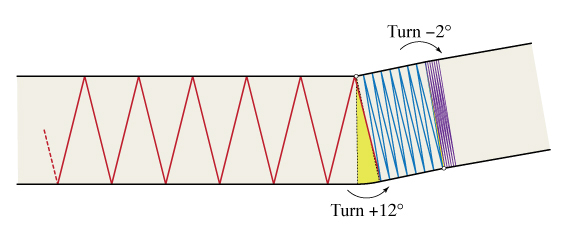Here is the phase portrait of $(\theta, x \; \mathrm{mod} \; 2)$ for the rays that Dimitri suggested in his response to Q2 (if I have interpreted his suggestion correctly), using the same data as displayed in the histogram:Following Dimitri's second suggestion in the comment below, I shot the ray off at a random start angle, e.g.: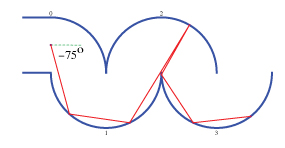Here are the phase portraits of 20 different random rays, with initial angles $\theta_0$ chosen uniformly within $(-\pi/2,\pi/2)$, each reflected through a tube of 200 semicircles. Each ray's phase trajectory is in a different color. The conventions are the same as in the phase portrait above (which corresponds to $\theta_0=0$). (Note: The number of ray reflections is not held constant, but rather the length of the tube is fixed, and the ray bounces until it exits.)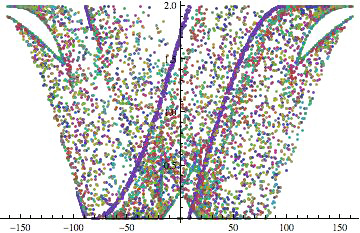I believe some of the prominently dark bands are caused by $\theta_0 \approx -\pi/2$, when the ray essentially skims around semicircle 1 and then shoots off nearly vertical to hit semicircle 2, and then it again nearly follows semicircle 3, and so on. You can see a hint of this behavior in the $\theta_0=-75^\circ$ example above.

• Very interesting... This trajectory looks very much like quasi-periodic. I was suspecting on the contrary that the situation is generic and the trajectory will fill a two-dimensional domain... If you are interested to play more with this billiard it would be interesting to plot some other trajectories (with different starting data). Just for sake of fun. Some of other trajectories should probably fill two-dimensional domains rather than sit on a smooth curve. This happen for generic trajectories in non-integrable billiards, and I would think that your billiard is non-integrable. Jul 16, 2011 at 0:20
• @Dmitri, not to be demeaning, but I'm not sure why periodicity at some level would be a surprise here. Jul 16, 2011 at 2:17
• Andrew, sure, some levels can be quasi-periodic, and they even constitute a non-zero measure inside of the set of all trajectories provided the boundary of the billiard is smooth -- by KAM. But just I was thinking that the first chosen trajectory will be chaotic :) . By the way, in reality we can not be 100 % sure that the first trajectory is quasi-periodic before we prove it... Jul 16, 2011 at 8:18

I would like to add one more piece of information and some pictures concerning the snake like tube. All this was communicated to me by Peter Panov.

To analyze the movement of a ray in the tube we will study instead the movement of a ray in the billiard depicted below. To get the billiard from the tube one should cut from the tube a piece included between two vertical segments of length 1 passing through two neighboring "cusps" of the tube.

The picture below shows one particular trajectory in the billiard.Every trajectory of a light ray inside the tube can be obtained by "developing" the corresponding trajectory in the billiard.

To analyse the billiard we will construct its phase portrait using canonical coordinates. The first coordinate is the distance along the boundary of the billiard from its up-most corner to the place were the ray hits the billiard's boundary. This distance is counted counter-clockwise and varies from $0$ to $2+\pi$ ($2+2\pi$ is the perimeter of the billiard). The second coordinate is the cosine of the angle between the trajectory of the reflected ray and the boundary of the billiard. This coordinate varies from $-1$ to $1$.

The picture below shows one particular trajectory in the phase space.The next picture shows the full phase portrait.To construct this phase portrait $19$ trajectories perpendicular to the left segment of the billiard were used. These trajectories correspond to red points in the picture. After this $19$ trajectories perpendicular to the right segment were constructed and they correspond to blue points on this picture. To get the actual picture both blue and red points were undergone small stochastic perturbations, and the perturbation for red points was slightly larger than the perturbation of blue points. As a result we can clearly distinguish the trajectories that hit the right segment perpendicularly (they are red), and we can distinguish the trajectories that hit the left segment perpendicularly (they are blue). Finally, the central symmetric trajectories are red-blue.

• Beautiful phase portrait! It seems to me you may have enough for a nice article on this topic. Nov 20, 2015 at 15:44
• Wow, pardon the pun, but that's a nice illustration of the "butterfly effect" in billiards! May 1, 2016 at 5:34

Just a guess .. tend to think that all (in laser-like beam) rays entering the torus:

$$(c + a \cos \phi ) \cos\theta,(c + a \cos \phi ) \sin \theta,a \sin \phi )$$

remain inside of the twisted torus along geodesic trajectories, with angle of incidence and reflection being equal. Accordingly points projected inside the twisted torus remain inside for all values of $c$ in: $$(c + a \cos \phi ) \cos\theta,(c + a \cos \phi ) \sin \theta,a \sin \phi + c\, \theta )$$

when the infinitesimal arcs of geodesics are replaced by finite segments of tube. Assuming as per sketch $\pi c>a$ to avoid re-entry of beam after one turn of spiral tube.